# Prime numbers + reason - math problems

#### Number of examples found: 74

• Diofant equation250x + 120y = 5640
• DivisorsThe sum of all divisors unknown odd number is 2112. Determine sum of all divisors of number which is twice of unknown numbers.
• Year 2018The product of the three positive numbers is 2018. What are the numbers?
• Summands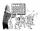Find two summands of the number 42, so that its product is minimized.
• Four-digit numberFind also a four-digit number, which quadrupled written backwards is the same number.
• NumberWhat number should be placed instead of the asterisk in number 702*8 to get a number divisible by 6?
• 7 digit numberIf 3c54d10 is divisible by 330, what is the sum of c and d?
• Six-digit primesFind all six-digit prime numbers that contain each one of digits 1,2,4,5,7 and 8 just once. How many are they?
• Unknown integerFind the smallest integer that: divided by 2, the remainder is 1 divided by 3 the remainder is 2, divided by 4 remainder is 3, ... divided by eight reminder is 7, by 9 reminder is 8.
• Twenty-fiveHow many three-digit natural numbers are divisible by 25?
• Z7-I-4 stars 4949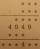Write instead of stars digits so the next write of product of the two numbers to be valid: ∗ ∗ ∗ · ∗ ∗ ∗ ∗ ∗ ∗ ∗ 4 9 4 9 ∗ ∗ ∗ ∗ ∗ ∗ 4 ∗ ∗
• Lines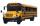Five bus lines runs at intervals 3, 6, 9, 12, 15 minutes. In the morning, suddenly start at 4:00. After how many minutes the bus lines meet again?
• RectanglesHow many different rectangles can be made from 60 square tiles of 1 m square? Find the dimensions of these rectangles.
• The King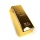The King wants to divide his sons equally. He has 42 rubies and 45 diamonds. How many sons and how will they share them?
• Chocolate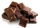I have a box of chocolate - white, milk and dark. The ratio of white to milk with dark is 3: 4. The ratio of white and milk to dark is 17: 4. Calculate what is the ratio between white, milk, dark chocolate.
• Dance groupThe dance group formed groups of 4, 5, and 6 members. Always one dancer remains. How many dancers were there in the whole group?
• Reminder and quotientThere are given the number C = 281, D = 201. Find the highest natural number S so that the C:S, D:S are with the remainder of 1,
• Divisibility by 12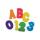Replace the letters A and B by digits so that the resulting number x is divisible by twelve /find all options/. x = 2A3B How many are the overall solutions?
• Florist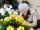Florist has 84 red and 48 white roses. How many same bouquets can he make from them if he must use all roses?
• TilesHow many tiles of 20 cm and 30 cm can build a square if we have a maximum 100 tiles?

Do you have an interesting mathematical word problem that you can't solve it? Submit a math problem, and we can try to solve it.

We will send a solution to your e-mail address. Solved examples are also published here. Please enter the e-mail correctly and check whether you don't have a full mailbox.

Please do not submit problems from current active competitions such as Mathematical Olympiad, correspondence seminars etc...